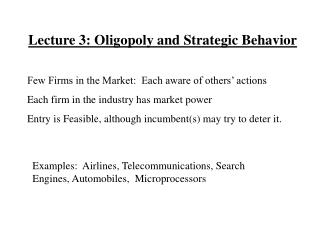DownloadDownload PresentationLecture 3: Oligopoly and Strategic Behavior

# Lecture 3: Oligopoly and Strategic Behavior

Download Presentation## Lecture 3: Oligopoly and Strategic Behavior

- - - - - - - - - - - - - - - - - - - - - - - - - - - E N D - - - - - - - - - - - - - - - - - - - - - - - - - - -
##### Presentation Transcript

1. Lecture 3: Oligopoly and Strategic Behavior Few Firms in the Market: Each aware of others’ actions Each firm in the industry has market power Entry is Feasible, although incumbent(s) may try to deter it. Examples: Airlines, Telecommunications, Search Engines, Automobiles, Microprocessors

2. Measuring market concentration: concentration indices Ci = combined market share of i largest firms, eg, C4. H = Herfindahl index, aka HHI. H = åsi2 both of these vary between 0 (many, many firms) and 1 (monopoly). Example: 4 firms in the market: S1=.50, S2=.25, S3=.15, S4=.10 C3 = .90, HHI= .52+.252+.152 + .102 =.345

3. Market structure and performance Nature of comp. Value of H Intensity of price comp. perfect below .2 fierce oligopoly .2 to .6 varies* monopoly .6 or above light *“Firms must understand the nature of markets in which they compete. There are reasons why, for example, even firms in an industry such as pharmaceuticals have, by economywide standards, impressive profitability, while top firms in the airline industry seem to achieve low rates of profitability even in the best of times.” (BDS, p.7)

4. In an oligopoly, degree of market power depends on: Demand Elasticity Market Concentration Collusive Behavior The “five forces framework” (Michael Porter, Competitive Strategy, NY, Free Press, 1980) Degree of market power depends primarily on rivalry within the industry, but also takes into account, vertical structure, the threat of entry, and threat of substitute products.

5. Quantity (Cournot) Competition Assume Homogeneous Products: MC=10 for both firms (Results are qualitatively similar with differentiated products) Demand: P=70-Q= 70- (q1+q2), since Q= q1+q2, where Q=total quantity. p1= Pq1 – TC1= (70-q1-q2) q1 -10q1 p1 =60q1 – q12 – q2q1 dp1/dq1=60-2q1-q2 =0 or q1=(60-q2)/2 (Reaction function of firm 1) Similarly, p2 =60q2 – q22 – q1q2 q2=(60-q1)/2 (Reaction function of firm 2)

6. Reaction function of firm 1 q2 Reaction function of firm 2 q1 Solve for the Nash Equilibrium Quantities Reaction functions intersect at q1= q2=20. Nash equilibrium is (q1*=20, q2*=20). P=30

7. Computing Profits: p1= p2 = (P-MC)q= (30-10)20=400. What would a monopoly do? MR=MC implies that 70-2Q=10, or Q=30. P=40 and total profits are 900. If each firm produced q=15, each firm would earn 450. Prices are higher and total quantity is lower under monopoly. Suppose that there were three firms. Total quantity would exceed 40 and the price and the profits would fall. As the number of firms gets very large, “Cournot” model approaches perfect competition. (Work out details!)

8. Oligopoly Models and Repeated Interaction: Example Let firm 1=SA, firm 2=Rest of the Cartel RC. Product is oil Suppose that the strategies of both SA and RC are limited to either producing 20 million barrels or 15 million barrels. If one firm produces 20 million barrels and the other firm produces 15 million barrels, P=35 and the firm producing 20 million barrels earns 500, while the firm producing 15 million barrels earns 375.

9. A one shot game Rest of Cartel’s Output 15 20 450 500 15 Saudi Arabia’s Output 450 375 375 400 20 500 400 Unique Nash equilibrium of one shot game is (20,20)

10. Repeated Games Suppose that each firm adopts the following Tit for Tat “trigger” strategy: I will produce at the “cooperative” level as long as my competitor did so if the previous period. If, however, my competitor deviates from that level, I will produce 20 million barrels forever. (Punishment threats must be credible to be effective.) Each player must determine whether it is worthwhile to deviate from the cooperative output level. Such a deviation results in a short term gain. But there are long term losses. Hence there is a tradeoff which depends, in part, on the discount rate.

11. Cooperation yields the following payoff: 450+ 450/(1+r) + 450/(1+r)2 +…. = 450(r +1)/r Deviation yields the following payoff 500+ 400/(1+r) + 400/(1+r)2 +….= 500+ 400/r Cooperation can be sustained if: r<(450-400)/(500-450)=1, that is if the discount rate is less than 100%. In general cooperation can be sustained if r<(cartel profit – one shot eq. Profit)/(deviation profit - cartel profit).

12. Without repetition of play, players are less likely to cooperate Repetition can create much stronger incentives to cooperate Trading off the gains from being non-cooperative today with the last future cooperation Tradeoff of SR gains vs. LR losses means that the discount rate matters